Next: Robot singularity analysis Up: Examples of applications of Previous: A robot kinematics example   Contents

A robot control problem

The problem presented here is for a robot described in the previous section. We have seen that the hand of the robot was moving with respect to its base by using linear actuators that allow to change the distance between the pairs of points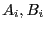. But in practice these actuators have a limited stroke and consequently the distance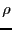between the points has to lie within a given range: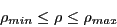Furthermore the actuator is attached at pointby joints (typically ball-and-socket or universal joints) that have limited motion. Hence the platform may reach only a limited set of position/orientation that is called its workspace.

Assume now that the hand has to follow a time-dependent trajectory: it is clearly important to verify that all the points of this trajectory lie within the workspace of the robot. The trajectory is defined in the following manner: the position/orientation parameter are written as analytic function of the time T (which is assumed to lie in the range [0,1] without lack of generality). Using the solution of the inverse kinematics (see the previous section) it is then possible to express the distanceas functions of the time. For the trajectory to lie in the workspace we have to verify the 12 inequalities: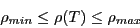when T lie in the range [0,1]. As the analytical form of the position/orientation parameters may be arbitrary we are looking for a generic algorithm that may deal with such arbitrary trajectory.

This can easily be done with interval analysis (see  for a detailed version). First we define the trajectory in Maple and compute the analytical form of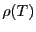(and of any other constraint that may limit the workspace of the robot). We get a set of inequalities that has to be satisfied for any T in [0,1] if the trajectory lie within the workspace. The analytical form of these inequalities are written in a file: this allow their interval evaluation for any T range by using the ALIAS parser. Then the general solving procedure of ALIAS may be used to determine if there is a T such that at least one constraint is violated.

An important point is that the algorithm allow to deal with the uncertainties in the problem. A first uncertainty occurs when controlling the robot along its nominal trajectory. Indeed the robot controller is not perfect and there will be a positioning error: for a nominal value of the position/orientation parameters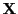the reached pose will be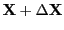where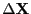can be bounded. A second uncertainty source is due to the differences between the theoretical geometrical model of the robot and its real geometry. Indeed to solve the inverse kinematics we use the coordinates of the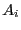in the reference frame and of the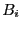in the model frame. In practice however these coordinates are known only up to a given accuracy: hence these coordinates for the real robot may have any value within given ranges. Hence the inequalities of the problem have not fixed value coefficients but interval coefficients. But this no problem for interval analysis as the general solving procedures may deal with such inequalities. Hence if the algorithm find out that all inequalities are verified for any T in [0,1], then this means that whatever is the real robot and the positioning error of the robot controller the trajectory followed by the robot will fully lie within the robot workspace.Next: Robot singularity analysis Up: Examples of applications of Previous: A robot kinematics example   Contents
Jean-Pierre Merlet 2012-12-20